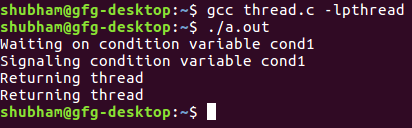Open In App

# Conditional wait and signal in multi-threading

What are conditional wait and signal in multi-threading?
Explanation: When you want to sleep a thread, condition variable can be used. In C under Linux, there is a function pthread_cond_wait() to wait or sleep.
On the other hand, there is a function pthread_cond_signal() to wake up sleeping or waiting thread.
Threads can wait on a condition variable.

```int pthread_cond_wait(pthread_cond_t *restrict cond,

Parameter :

```cond : condition variable
mutex : is mutex lock ```

Return Value :

```On success, 0 is returned ; otherwise, an error
number shall be returned to indicate the error. ```

The pthread_cond_wait() release a lock specified by mutex and wait on condition cond variable.

`int pthread_cond_signal(pthread_cond_t *cond);`

Parameter :

`cond : condition variable`

Return Value :

```On success, 0 is returned ; otherwise, an error number
shall be returned to indicate the error. ```

Note : The above two functions works together.

Below is the implementation of condition, wait and signal functions.

## C

 `// C program to implement cond(), signal()``// and wait() functions``#include ``#include ``#include ` `// Declaration of thread condition variable``pthread_cond_t cond1 = PTHREAD_COND_INITIALIZER;` `// declaring mutex``pthread_mutex_t lock = PTHREAD_MUTEX_INITIALIZER;` `int` `done = 1;` `// Thread function``void``* foo()``{` `    ``// acquire a lock``    ``pthread_mutex_lock(&lock);``    ``if` `(done == 1) {` `        ``// let's wait on condition variable cond1``        ``done = 2;``        ``printf``(``"Waiting on condition variable cond1\n"``);``        ``pthread_cond_wait(&cond1, &lock);``    ``}``    ``else` `{` `        ``// Let's signal condition variable cond1``        ``printf``(``"Signaling condition variable cond1\n"``);``        ``pthread_cond_signal(&cond1);``    ``}` `    ``// release lock``    ``pthread_mutex_unlock(&lock);` `    ``printf``(``"Returning thread\n"``);` `    ``return` `NULL;``}` `// Driver code``int` `main()``{``    ``pthread_t tid1, tid2;` `    ``// Create thread 1``    ``pthread_create(&tid1, NULL, foo, NULL);` `    ``// sleep for 1 sec so that thread 1``    ``// would get a chance to run first``    ``sleep(1);` `    ``// Create thread 2``    ``pthread_create(&tid2, NULL, foo, NULL);` `    ``// wait for the completion of thread 2``    ``pthread_join(tid2, NULL);` `    ``return` `0;``}`

Output:Time Complexity:
The time complexity of this program is O(1), as the program only requires one iteration of each thread.

Space Complexity:
The space complexity of this program is O(1), as the program only requires a few variables to be stored in memory.